# Statistics - Standard Least Squares Fit (Gaussian linear model)

least square is a regression method.

In a least squares, the coefficients are found in order to make RSS as small as possible.

When p is be much bigger than n (the number of samples), we can't use full least squares, because the solution's not even defined.

Legendre published the method of least squares in 1805.

## Scale-invariant

Standard least squares is scale-invariant The scaling of the variable doesn't matter because if a feature is multiplied by a constant, the coefficient can be divided by the same constant in order to get the same target.

Whether a length is measured in feet or inches is not going to matter because the coefficient can just account for the change in units.

## Ordinary

Ordinary Least Square (OLS) is a least square procedure performs on the the raw predictors.

## Documentation / Reference

Recommended Pages(Machine learning|Inverse problems) - Regularization

Regularization refers to a process of introducing additional information in order to: solve an ill-posed problem or to prevent overfitting. This information is usually of the form of a penalty...(Prediction|Recommender System) - Collaborative filtering

Collaborative filtering is a method of making automatic predictions (filtering) the interests of a user by collecting preferences or taste information from many users (collaborating). But in general,...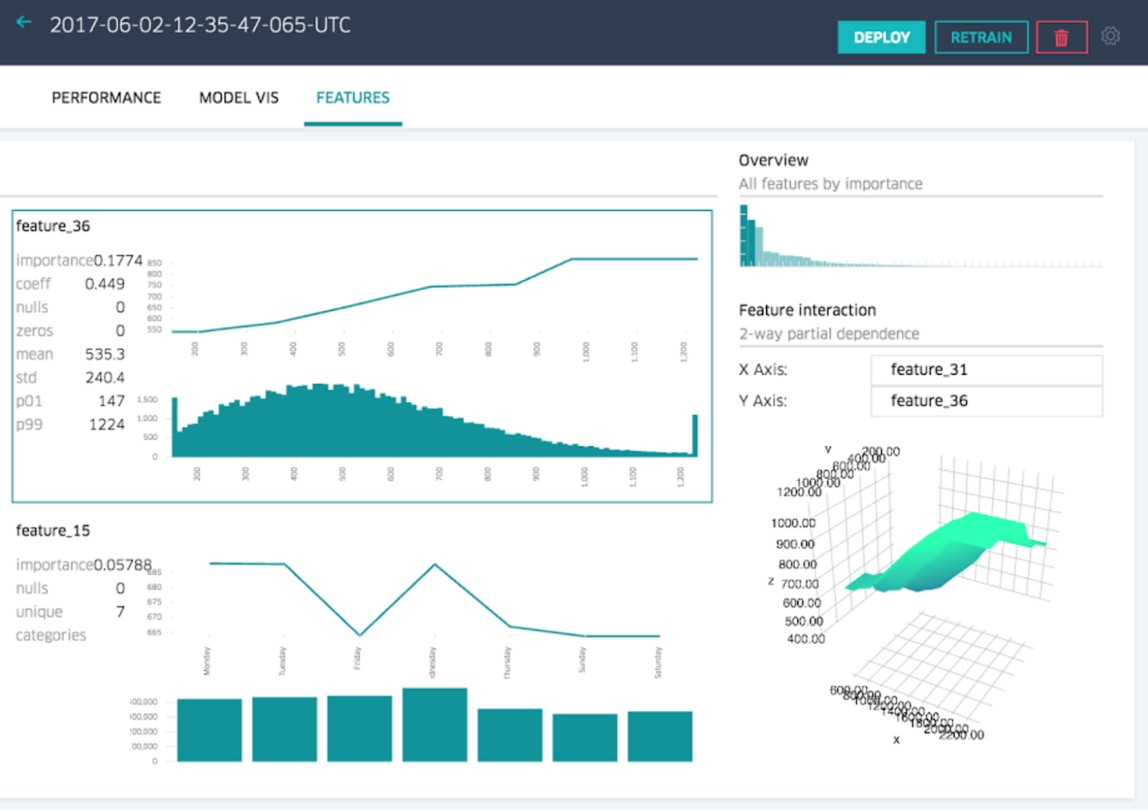Data Mining - (Attribute|Feature) (Selection|Importance)

Feature selection is the second class of dimension reduction methods. They are used to reduce the number of predictors used by a model by selecting the best d predictors among the original p predictors....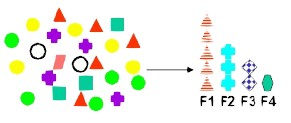Data Mining - (Feature|Attribute) Extraction Function

Feature extraction is the second class of methods for dimension reduction. dimension reduction It creates new attributes (features) using linear combinations of the (original|existing) attributes. ...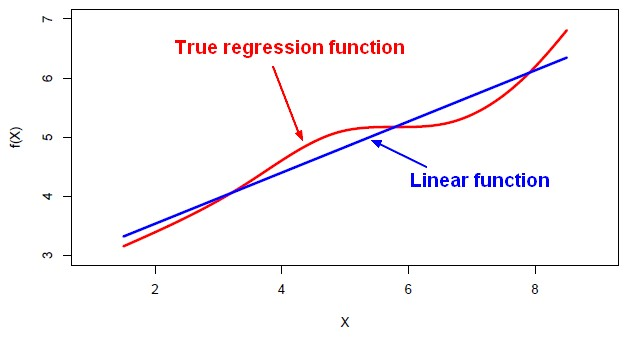Machine Learning - Linear (Regression|Model)

Linear regression is a regression method (ie mathematical technique for predicting numeric outcome) based on the resolution of linear equation. This is a classical statistical method dating back more...Notion - (Best approximation|Closest|Closeness)

This notion of “best approximates” comes up again and again: in least-squares, a fundamental data analysis technique, image compression, in principal component analysis, another data analysis...R - K-fold cross-validation (with Leave-one-out)

Cross-validation in R. Leave-one-out cross-validation in R. Each time, Leave-one-out cross-validation (LOOV) leaves out one observation, produces a fit on all the other data, and then makes a...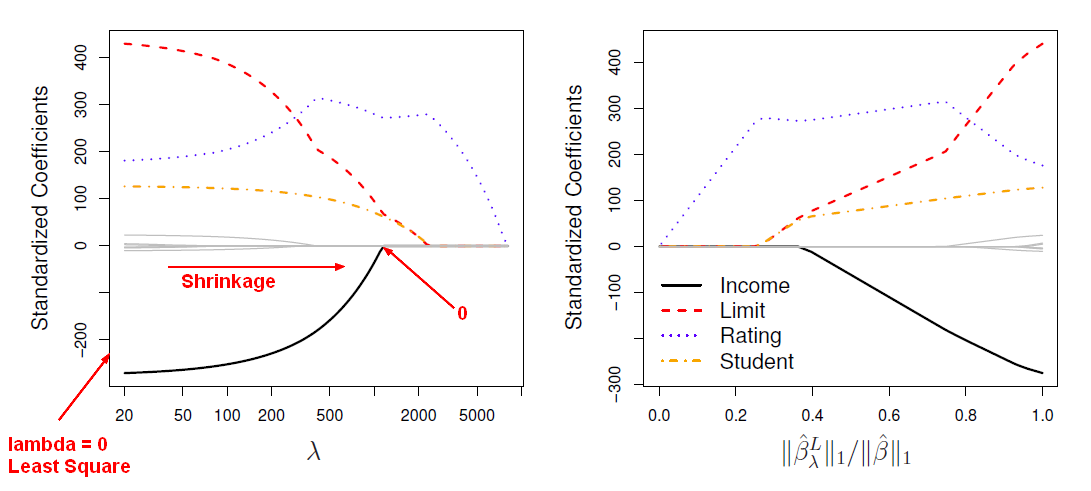Statistical Learning - Lasso

Lasso is a shrinkage method. Ridge regression doesn't actually select variables by settings the parameters to zero. Lasso is a more recent technique for shrinking coefficients in regression that overcomes...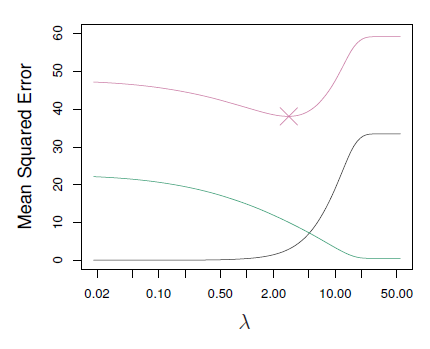Statistics - (Shrinkage|Regularization) of Regression Coefficients

Shrinkage methods are more modern techniques in which we don't actually select variables explicitly but rather we fit a model containingall p predictors using a technique that constrains or regularizes...